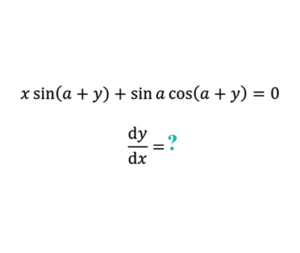Home -> Solved problems -> Find the derivative of y with respect to x

## Find the derivative of $$y$$ with respect to $$x$$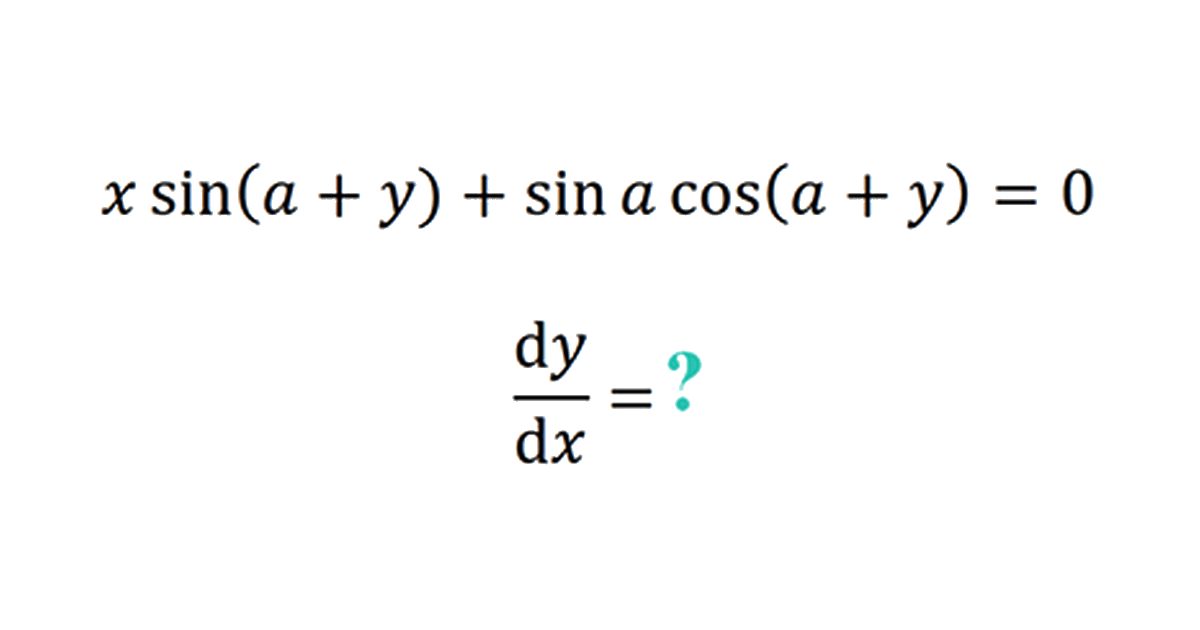### Solution

\begin{aligned} &\mathrm{d}(x \sin (a+y)+\sin a \cos (a+y))=\mathrm{d}(0)=0 \\\\ &\mathrm{~d}(x \sin (a+y))+\sin a d(\cos (a+y))=0 \\\\ &\sin (a+y) d x+x \mathrm{~d}(\sin (a+y))+\sin a \mathrm{~d}(\cos (a+y))=0 \end{aligned}
\begin{aligned} &\sin (a+y) d x+x(\cos (a+y)) d y-\sin a \sin (a+y) d y=0 \\\\ &\sin (a+y) d x+(x \cos (a+y)-\sin a \sin (a+y)) d y=0 \\\\ &\Rightarrow \frac{\mathrm{d} y}{\mathrm{~d} x}=\frac{\sin (a+y)}{\sin a \sin (a+y)-x \cos (a+y)} \\\\ &=\frac{\sin (a+y)}{\sin a \sin (a+y)+\cos (a+y)\left(\frac{\sin a \cos (a+y)}{\sin (a+y)}\right)} \\\\ &=\frac{\sin (a+y)}{\sin a \sin (a+y)+\left(\frac{\sin a \cos ^{2}(a+y)}{\sin (a+y)}\right)} \end{aligned}
\begin{aligned} &=\frac{\sin ^{2}(a+y)}{\sin a \sin ^{2}(a+y)+\sin a \cos ^{2}(a+y)} \\\\ &=\frac{\sin ^{2}(a+y)}{\sin a\left(\sin ^{2}(a+y)+\cos ^{2}(a+y)\right)} \end{aligned} $\huge \Rightarrow \frac{d y}{d x}=\frac{\sin ^{2}(a+y)}{\sin a}$
Home -> Solved problems -> Find the derivative of y with respect to x

### Related Topics

Find the volume of the square pyramid as a function of $$a$$ and $$H$$ by slicing method.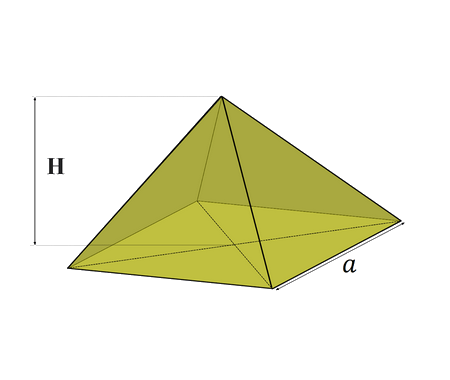Prove that $\lim_{x \rightarrow 0}\frac{\sin x}{x}=1$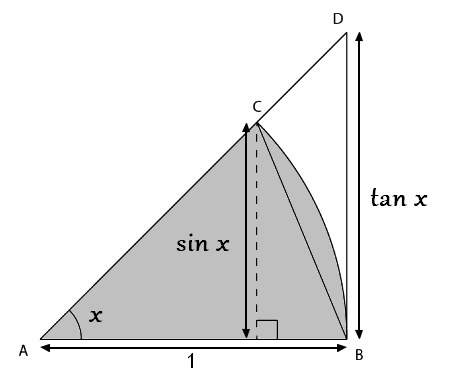Prove that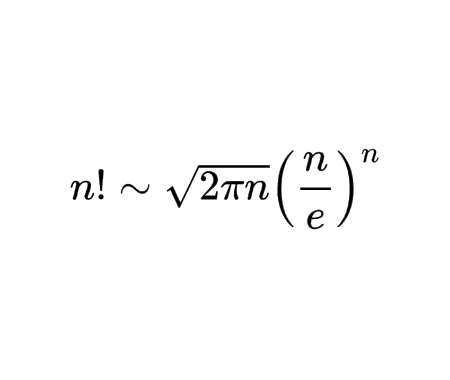Calculate the half derivative of $$x$$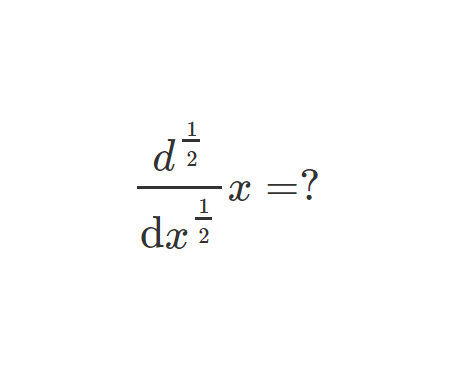Prove Wallis Product Using Integration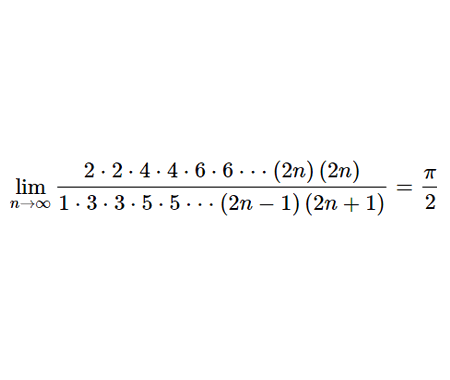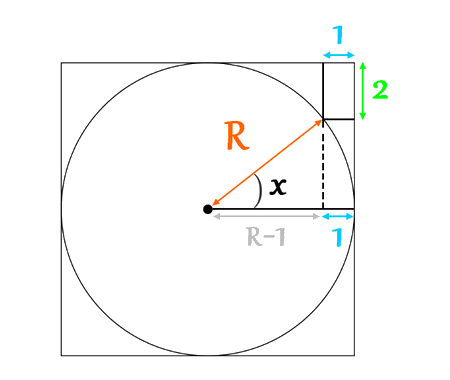Calculate the volume of Torus using cylindrical shells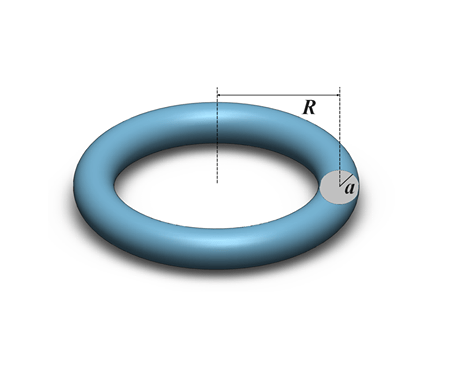Find the derivative of exponential $$x$$ from first principles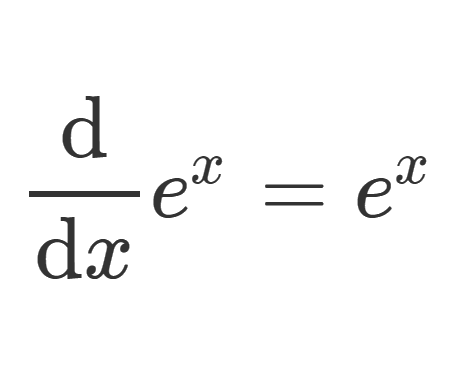Calculate the sum of areas of the three squares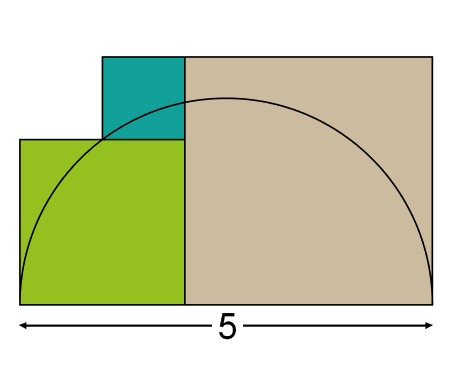Find the equation of the curve formed by a cable suspended between two points at the same height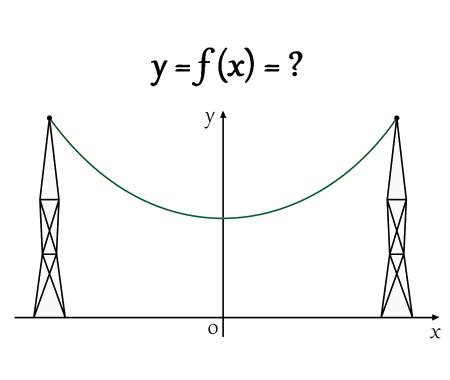Solve the equation for real values of $$x$$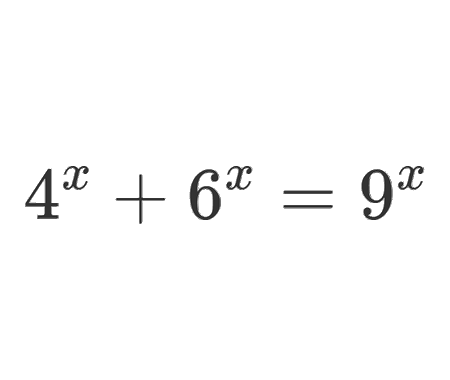Solve the equation for $$x\epsilon\mathbb{R}$$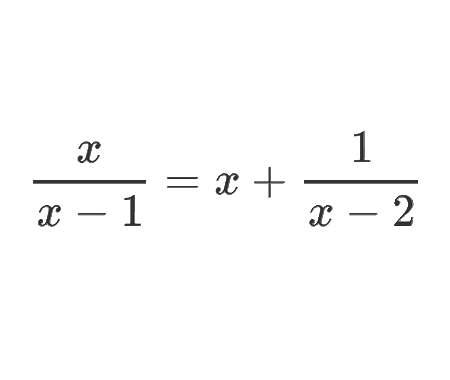Determine the angle $$x$$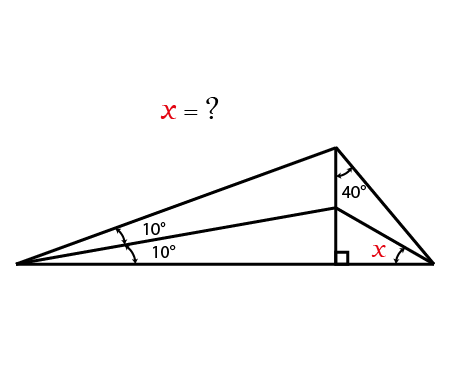Calculate the following limit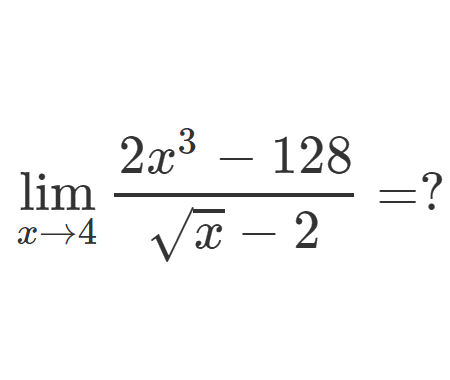Calculate the following limit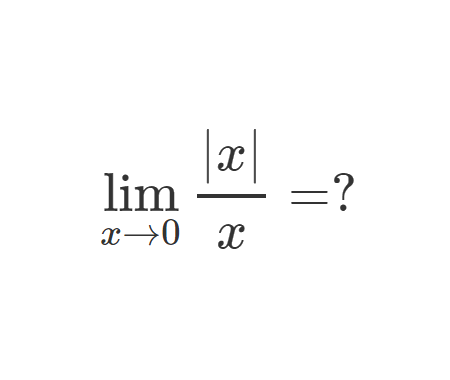Calculate the integral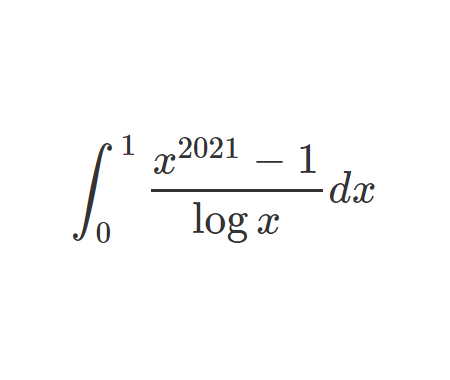Challenging problem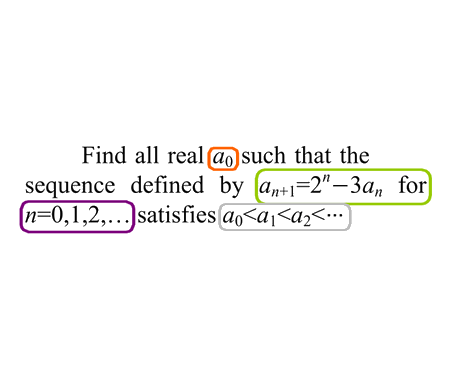Prove that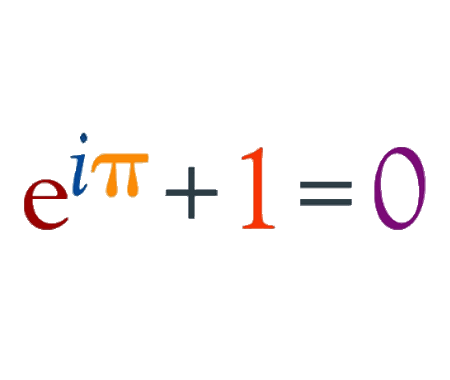Prove that $$e$$ is an irrational numberFind the derivative of $$y$$ with respect to $$x$$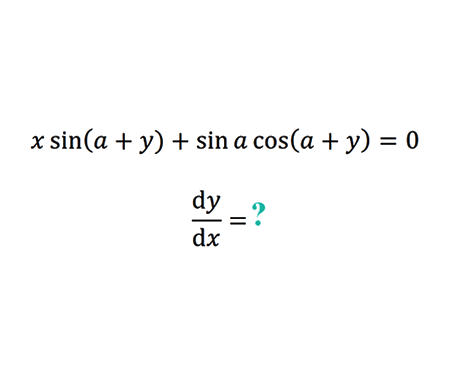Home -> Solved problems -> Find the derivative of y with respect to x

#### Share the solution: Find the derivative of y with respect to x